Test: Magnetostatics

# Test: Magnetostatics

Test Description

## 20 Questions MCQ Test GATE Electrical Engineering (EE) 2023 Mock Test Series | Test: Magnetostatics

Test: Magnetostatics for Railways 2023 is part of GATE Electrical Engineering (EE) 2023 Mock Test Series preparation. The Test: Magnetostatics questions and answers have been prepared according to the Railways exam syllabus.The Test: Magnetostatics MCQs are made for Railways 2023 Exam. Find important definitions, questions, notes, meanings, examples, exercises, MCQs and online tests for Test: Magnetostatics below.
Solutions of Test: Magnetostatics questions in English are available as part of our GATE Electrical Engineering (EE) 2023 Mock Test Series for Railways & Test: Magnetostatics solutions in Hindi for GATE Electrical Engineering (EE) 2023 Mock Test Series course. Download more important topics, notes, lectures and mock test series for Railways Exam by signing up for free. Attempt Test: Magnetostatics | 20 questions in 60 minutes | Mock test for Railways preparation | Free important questions MCQ to study GATE Electrical Engineering (EE) 2023 Mock Test Series for Railways Exam | Download free PDF with solutions
 1 Crore+ students have signed up on EduRev. Have you?
Test: Magnetostatics - Question 1

### A rectangular loop of wire in free space joins points A(1, 0, 1) to B(3, 0, 1) to C(3, 0, 4) to D(1, 0, 4) to A. The wire carries a current of 6 mA flowing in the uz direction from B to C. A filamentary current of 15 A flows along the entire z, axis in the uz directions. Que: The force on side BC is

Detailed Solution for Test: Magnetostatics - Question 1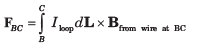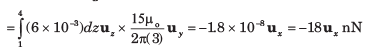Test: Magnetostatics - Question 2

### A rectangular loop of wire in free space joins points A(1, 0, 1) to B(3, 0, 1) to C(3, 0, 4) to D(1, 0, 4) to A. The wire carries a current of 6 mA flowing in the uz direction from B to C. A filamentary current of 15 A flows along the entire z, axis in the uz directions. Que: The force on side AB is

Detailed Solution for Test: Magnetostatics - Question 2

The field from the long wire now varies with position along the loop segment.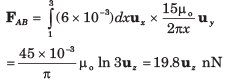Test: Magnetostatics - Question 3

### A rectangular loop of wire in free space joins points A(1, 0, 1) to B(3, 0, 1) to C(3, 0, 4) to D(1, 0, 4) to A. The wire carries a current of 6 mA flowing in the uz direction from B to C. A filamentary current of 15 A flows along the entire z, axis in the uz directions. Que: The total force on the loop is

Detailed Solution for Test: Magnetostatics - Question 3

This will be the vector sum of the forces on the four sides. By symmetry, the forces on sides AB and CD will be equal and opposite, and so will cancel. This leaves the sum of forces on side BC and DA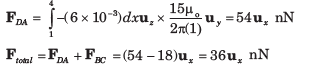Test: Magnetostatics - Question 4

Consider the rectangular loop on z = 0 plane shown in fig. The magnetic flux density is B = 6 xux - 9 yuy + 3zuz Wb/m2. The total force experienced by the rectangular loop is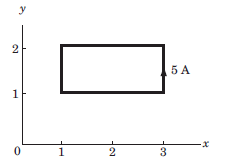Detailed Solution for Test: Magnetostatics - Question 4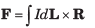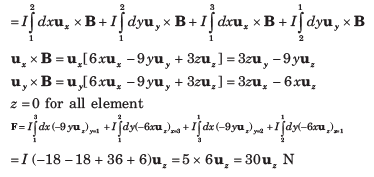Test: Magnetostatics - Question 5

Three uniform current sheets are located in free space as follows: 8uz A/m at y = 0, -4uz A/m at y = 1 and -4uz A/m at y = -1. Let F be the vector force per meter length exerted on a current filament carrying 7 mA in the uL direction.

Que: If the current filament is located at x = 0, y = 0.5 andu uL =  uZ , then F is

Detailed Solution for Test: Magnetostatics - Question 5

Within the region 1 < y <1, the magnetic fields from the two outer sheets (carrying -4 uz A/m) cancel, leaving only the field from the center sheet. Therefore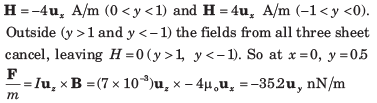Test: Magnetostatics - Question 6

Three uniform current sheets are located in free space as follows: 8uz A/m at y = 0, -4uz A/m at y = 1 and -4uz A/m at y = -1. Let F be the vector force per meter length exerted on a current filament carrying 7 mA in the uL direction.

Que: If the current filament is located at y = 0.5, z = 0, and uL = ux, then F is

Detailed Solution for Test: Magnetostatics - Question 6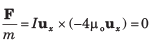Test: Magnetostatics - Question 7

Two infinitely long parallel filaments each carry 100 A in the uz direction. If the filaments lie in the plane y = 0 at x = 0 and x = 5 mm, the force on the filament passing through the origin is

Detailed Solution for Test: Magnetostatics - Question 7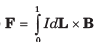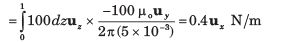Test: Magnetostatics - Question 8

A conducting current strip carrying K =  6uz A/m lies in the x = 0 plane between y = 0.5 and y = 1.5 m. There is also a current filament of I = 5 A in the uz direction on the z –axis.

Que: The force exerted on the filament by the current strip is

Detailed Solution for Test: Magnetostatics - Question 8

The field from the current strip at the filament location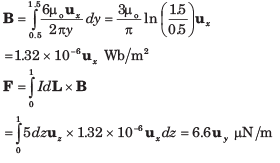Test: Magnetostatics - Question 9

A conducting current strip carrying K =  6uz A/m lies in the x = 0 plane between y = 0.5 and y = 1.5 m. There is also a current filament of I = 5 A in the uz direction on the z –axis.

Que: The force exerted on the strip by the filament is

Detailed Solution for Test: Magnetostatics - Question 9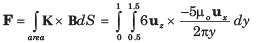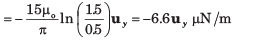Test: Magnetostatics - Question 10

In a material the magnetic field intensity is H = 1200A/m when B = 2 Wb/m2. When H is reduced to 400 A/m, B =1.4 Wb/m2. The change in the magnetization M is

Detailed Solution for Test: Magnetostatics - Question 10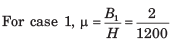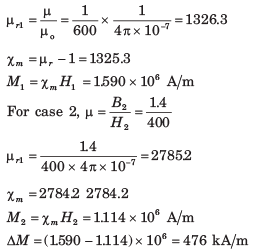Test: Magnetostatics - Question 11

In a certain material for which μr = 6.5 ,

H = 10ux + 25uy - 40uz  A/m

Que:

The magnetic susceptibility χm of the material is

Detailed Solution for Test: Magnetostatics - Question 11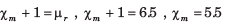Test: Magnetostatics - Question 12

In a certain material for which μr = 6.5 , H = 10ux + 25uy - 40uz  A/m

Que: The magnetic flux density B is

Detailed Solution for Test: Magnetostatics - Question 12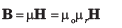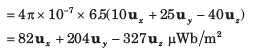Test: Magnetostatics - Question 13

In a certain material for which μr = 6.5 , H = 10ux + 25uy - 40uz  A/m

Que: The magnetization M is

Detailed Solution for Test: Magnetostatics - Question 13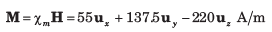Test: Magnetostatics - Question 14

In a certain material for which μr = 6.5 , H = 10ux + 25uy - 40uz  A/m

Que: The magnetic energy density is

Detailed Solution for Test: Magnetostatics - Question 14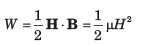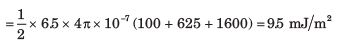Test: Magnetostatics - Question 15

For a given material magnetic susceptibility χm = 3.1 and within which B = 0.4 yuz T.

Que: The magnetic field H is

Detailed Solution for Test: Magnetostatics - Question 15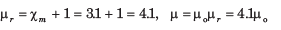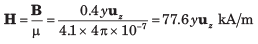Test: Magnetostatics - Question 16

For a given material magnetic susceptibility χm = 3.1 and within which B = 0.4 yuz T.

Que: The magnetization M is

Detailed Solution for Test: Magnetostatics - Question 16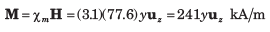Test: Magnetostatics - Question 17

A particular material has 2.7 x 1029  atoms/m3 and each atom has a dipole moment of 2.6 x 1030 uy A .m2. The H in material is (μr = 4.2 )

Detailed Solution for Test: Magnetostatics - Question 17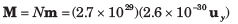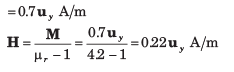Test: Magnetostatics - Question 18

In a material magnetic flux density is 0.02 Wb/mand the magnetic susceptibility is 0.003. The magnitude of the magnetization is

Detailed Solution for Test: Magnetostatics - Question 18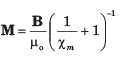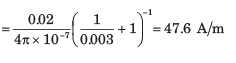Test: Magnetostatics - Question 19

A uniform field H = -600 uy A/m exist in free space. The total energy stored in spherical region 1 cm in radius centered at the origin in free space is

Detailed Solution for Test: Magnetostatics - Question 19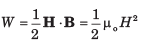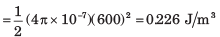Test: Magnetostatics - Question 20

The magnetization curve for an iron alloy is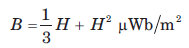approximately given by If H increases from 0 to 210 A/m, the energy stored per unit volume in the alloy is

Detailed Solution for Test: Magnetostatics - Question 20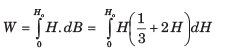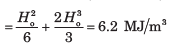## GATE Electrical Engineering (EE) 2023 Mock Test Series

22 docs|274 tests
 Use Code STAYHOME200 and get INR 200 additional OFF Use Coupon Code